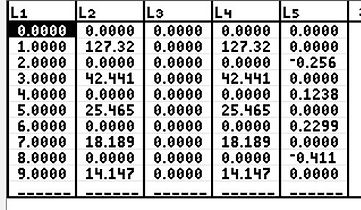naver-site-verification: naver47238a1b6bfbb19a2fd4b619734fa9a6.html
top of page

# Fourier analysis  with the Ti-84 plus (CE)

## Spectrum Analysis (Harmonics) of Periodic Signals

In Electrical Engineering, the Fourier analysis is an important tool for analyzing the power factor and harmonics of currents and voltages. The programs FOURIER.8xp and FOURIERL.8xp calculate Fourier series of periodic signals very accurately and can be applied in combination with the signal builder program FUNCGEN.8xp.

The program FOURIER.8xp plots the result like a real spectrum analyzer, and the program FOURIERL.8xp gives more detailed results of the Fourier series, including amplitude and phase.

Fourier series are defined by the following formulas. According to the Fourier theory, every periodic function can be written as the sum of sine and cosine functions with multiple frequency which are relative to the basic frequency of the periodic signal. The following formulas are the basis of Ti-Basic programs FOURIER.8xp and FOURIERL.8xp.

## Defintions and formulas of The Fourier AnalysisThe first example is the output signal of a triac voltage regulator. The signal has been built by the program FUNCGEN. (Go to the page FUNGEN (signal builder) to read the manual of this program.From the signal above, the basic frequency equals 50 Hz, with a peak of 230√2=325.27V and a firing pulse on the gate of the triac at  90 and 270 degrees angles. Below, in the spectrum, calculated by the program Fourier.8xp, the first harmonic equals 192.7 V, a third harmonic of 103.53 V  (150 Hz) and a fifth harmonic of 34.52 V (250 Hz). These values agree with the analytical results.

This program requires some calculation time (some minutes) depending on the desired number of harmonics, so please be patient in order to obtain a plot. Faster calculations can be obtained with the program FOURIERL. A demonstration of this program is provided further on this page.## The Fourier program, calculating the amplitude and the phase of the harmonics

This program generates a list of the results.

If the Fourier series exists of an average value, sine part, cosine part  then:

F=A0 +A1sin(2πft)+B1cos(2πft)+A2sin(2π2ft)+B2cos(2π2ft)+A3sin(2π3ft)+B3cos(2π3ft)+.... or

F=A0+C1sin(2πft+ϕ1)+C2sin(2π2ft+ϕ2)+C3sin(2π3f+ϕ3)+......

L1(n)=number of harmonic , L2(0)=A0,  L2(n)=An, L3(n)=Bn, L4(n)=Cn, L5=fi(n)

The analysed input signal can be generated by FUNCGEN or defined directly, see the example.

In this example, the same signal is analysed as above.In the example, the same signal is analyzed as above. A sine function of 325.27V and firing angles of 90 and 270 degrees.  If we read the results from the lists, then it yields :

F=0+162.63*sin(2π50t)-103.5*cos(2π50t)+ 103.54 *cos(2π150t) -34,51cos(2π250t)  or F=0+192.79*sin(2π50t-32.48deg)+ 103.54*sin(2π150t+90deg)+34.512sin(2π250t-90deg) + 34.512sin(2π250t+90deg)

Another example of the Fourier analysis of the well known square wave (amplitude 100 V and base frequency of 100Hz.) The square wave is built with the signal builder program FUNCGEN.8xp

### Fourier Analysis of the Square waveFor the nth harmonic yields  = (4/nπ)* 100. This results for the first harmonic: 127.32,  third harmonic 42.441 and for the fifth: 25.4646 etc. Conclusion. The results of the program are in very good agreement  ith the analytical results

### The Fourier analysis of a triangle function

At the end, the Fourier analysis program calulates the harmonics of a triangle function with an average value. With the help of the program Funcgen the triangle function has been constructed with a period time of 1/120 sec equals 120 Hz and an amplitude of 25 with an average value of 12.5For the program FOURIER.8xp , FOURIERL.8xp and FUNCGEN send a request to frieboon@gmail.com or chat and the program will be sent.